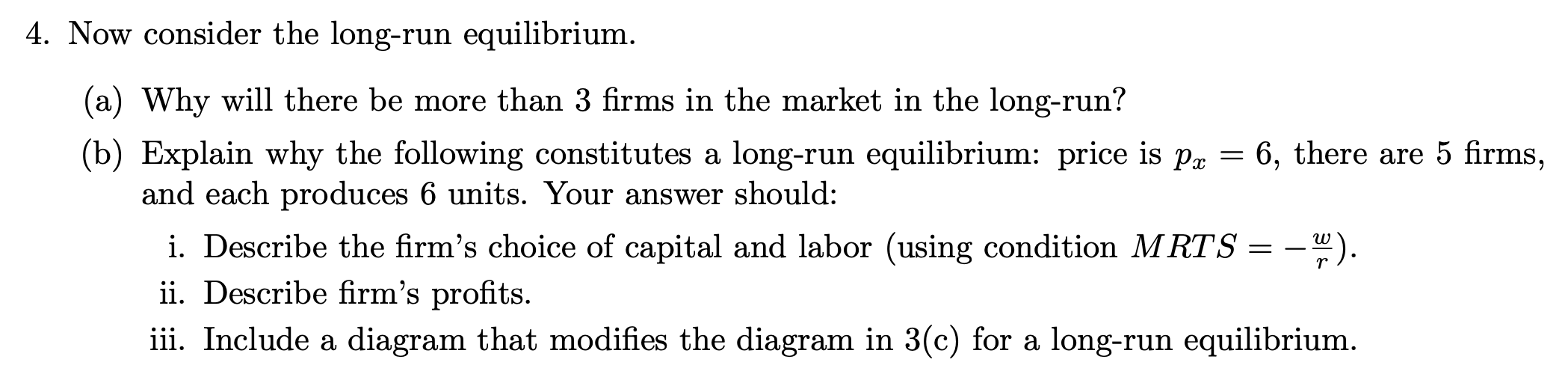### Create an Account

Home / Questions / Given the following information, please answer the following question

The long run production function for each firm is 2K^0.5L^0.5

The wage rate is w = 18, the rental rate of capital is r = 2, and K is fixed in the short-run at K = 9.4. Now consider the long-run equilibrium (a) Why will there be more than 3 firms in the market in the long-run? (b) Explain why the following constitutes a long-run equilibrium: price is p and each produces 6 units. Your answer should: 6, there are 5 firms, i. Describe the firm&#39;s choice of capital and labor (using condition MRTS ii. Describe firm&#39;s profits. i. Include a diagram that modifies the diagram in 3(c) for a long-run equilibrium.

Feb 06 2020 View more View LessGet Solution